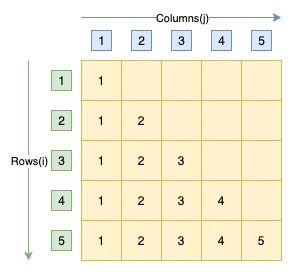# Java Program to Print Right Triangle Number Pattern

• Java program to print right triangle number pattern.

A right triangle number pattern contains N space separated consecutive natural numbers in Nth row.

Sample triangle pattern of 5 rows.
```1
1 2
1 2 3
1 2 3 4
1 2 3 4 5
```

Here is the matrix representation of the right triangle number pattern. The row numbers are represented by i whereas column numbers are represented by j.Algorithm to print right triangle number pattern
• There are N natural numbers in Nth Row. 1st row contains 1 number, 2nd row contains 2 numbers etc.
• In any row N, the numbers start from 1 and continue till N in increment of 1. For example, 4th row contains numbers from 1 to 4.
• We will use two for loops to print right triangle number pattern.
• For a right triangle number pattern of N rows, outer for loop will iterate N time(from i = 1 to N). Each iteration of outer loop will print one row of the pattern.
• The inner for loop will print all elements of a row. As we know that Nth row contains N elements from 1 to N. Hence, inner for loop will iterate from N times(j = 1 to N). Each iteration of inner for loop will print consecutive numbers starting from 1.

## Java program to print right triangle number pattern

```package com.tcc.java.programs;

import java.util.Scanner;

public class TrianglePattern {
public static void main(String[] arg) {
int rows, i, j;
Scanner scanner = new Scanner(System.in);
System.out.println("Enter Number of Rows of Pattern");
rows = scanner.nextInt();

/* Outer for loop prints one row in every iteration */
for (i = 1; i <= rows; i++) {
/* Inner for loop prints numbers of one row */
for (j = 1; j <= i; j++) {
System.out.print(j + " ");
}
System.out.print("\n");
}
}
}
```
Output
```Enter Number of Rows of Pattern
5
1
1 2
1 2 3
1 2 3 4
1 2 3 4 5
```